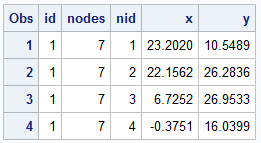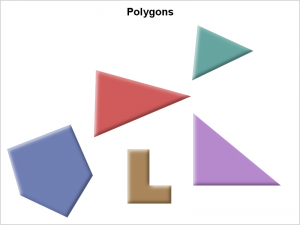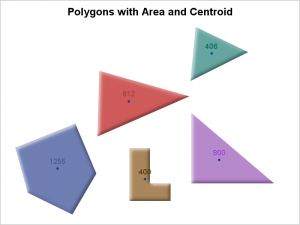A few weeks back I saw a couple of posts on the Communities page from users wanting to find ways to compute the area of an general polygon and also the center of the area.  I felt such features likely existed somewhere in the SAS/GRAPH set of procedures, so I asked our resident expert(s).  Initially, there was some miscommunication due to the requirement to compute areas.  The %Centroid macro is available among the Annotate macros but it does not report areas.  Also, see another clarification at the bottom of this article.In the meantime, this piqued my interest and I took a stab at it and wrote up a macro to compute the Area and the centroid of the polygon as described below.

First, I needed a few random polygons, so I wrote a small routine to generate the data in the format on the right.   Changing the seed values can generate different shapes, some concave.   I generated 3 polygons with random number of nodes, and  added one custom polygon to get a specific shapes like the right triangle and a concave "L" shape for verification.Then, I used the POLYGON statement in SAS 9.4 SGPLOT procedure to plot the polygons to see what I have, as shown on the right.  See code in the link below.

The macro is included in the code and takes a data set with columns shown above, along with the global XMin and YMin of the entire data (easily computed) and placed into a couple of macro variables XMin and YMin.  The macro takes the name of the input data set "DS", the xmin and ymin values previously computed (just to save a step in the macro to compute them), and polygon data with polygon "ID", and the coordinates.  The result is placed in the output data set "OUT".

%macro polyarea (ds=, xmin=, ymin=, out=, Id=, X=, Y=);

The macro steps around the polygon nodes and incrementally adds the segment areas of each parallelogram against both the X and Y axis.  The sum of each segment around X or Y should be (and are) equal.  Then it takes the first moment of the area of each parallelogram segment with its center about the XMin and YMin axes to compute the center of the area in each direction.  The "X" areas are used for computing the x coordinate, and "Y" areas for the y coordinate.The result is plotted with the SGPLOT polygon statement with a TEXT overlay to display the area and the location (x, y) of the center of area as shown on the right.  The results "look" kind of right to the eye.

I did not attempt to handle polygons with holes.  There is a good chance the algorithm itself will work for a polygon with holes.

I did not attempt to handle polygons with multiple segments, like in a map data sets.  This macro could be extended to get the area and CG of each segment by making each polygon have a unique id.  But to get the area of the composite multi-segment polygon would need some more thought.  That exercise is left to the motivated reader (meaning I am ducking that exercise).  :-).

If the polygon is highly concave, it is possible the CG will not be within the polygon boundary.  That would be another good exercise to think about.

I mentioned the %Centroid macro earlier.  Another difference between this macro and the %Centroid Macro is that this macro computes the mathematical centroid of each polygon, while the %Centroid macro computes a "good" location for labeling of a polygon, and not the mathematical centroid.  If you want to label a polygon (like state or country name), the %Centroid may be the preferred tool.

Area and Centroid Macro:  AreaCentroidMacro

ShareDirector, R&D

Sanjay Matange is R&D Director in the Data Visualization Division responsible for the development and support of the ODS Graphics system, including the Graph Template Language (GTL), Statistical Graphics (SG) procedures, ODS Graphics Designer and related software. Sanjay has co-authored a book on SG Procedures with SAS/PRESS.

### Related Posts

1.Jeff Phillips on

Thanks for sharing, Sanjay.

For what it's worth, there is a macro to calculate the centroid of map polygons as part of the annotation macros: annomac.

%annomac;
%centroid( input-map-data-set, output-data-set, list-of-ids );

It also takes an optional parameter "segonly=1" that only centers on the main polygon of a multipolygon set.

It handles irregularly shaped polygons (topologically correct though - no crossovers) and polygons with holes and multi-polygon sets.

2.I use integration by parts. It works for any non-self intersecting polygon (I hate those weirdos). If there is a hole in the polygon, then you just need to add in a polygon ID and a step to subtract that volume from the surrounding polygon.

DATA area(KEEP=area);
SET test END=last_point;
FORMAT area 6.2;
RETAIN prev_x prev_y start_x start_y area;
IF _N_ EQ 1 THEN DO;
prev_x = x; prev_y = y; start_x = x; start_y = y; area = 0.0;
END;
ELSE DO;
IF (x NE prev_x) THEN DO;
b = x - prev_x;
h1 = MIN(y, prev_y);
h2 = MAX(y, prev_y);
area = area + (b * h1) + (b * (h2 - h1) / 2);
END;
prev_x = x; prev_y = y;
END;
IF last_point EQ 1 THEN DO;
IF (start_x NE prev_x) THEN DO;
b = start_x - prev_x;
h1 = MIN(start_y, prev_y);
h2 = MAX(start_y, prev_y);
area = ABS(area +(b * h1) + (b * (h2 - h1) / 2));
END;
OUTPUT;
END;
ELSE DELETE;
RUN;